# On a new class of Hardy-type inequalities

## Abstract

In this paper, we generalize a Hardy-type inequality to the class of arbitrary non-negative functions bounded from below and above with a convex function multiplied with positive real constants. This enables us to obtain new generalizations of the classical integral Hardy, Hardy-Hilbert, Hardy-Littlewood-Pólya, and Pólya-Knopp inequalities as well as of Godunova’s and of some recently obtained inequalities in multidimensional settings. Finally, we apply a similar idea to functions bounded from below and above with a superquadratic function.

MSC: 26D10, 26D15.

## 1 Introduction

Let $0 and $\left({\mathrm{\Omega }}_{1},{\mathrm{\Sigma }}_{1},{\mu }_{1}\right)$, $\left({\mathrm{\Omega }}_{2},{\mathrm{\Sigma }}_{2},{\mu }_{2}\right)$ be measure spaces with positive σ-finite measures. Let $u:{\mathrm{\Omega }}_{1}\to \mathbb{R}$ and $k:{\mathrm{\Omega }}_{1}×{\mathrm{\Omega }}_{2}\to \mathbb{R}$ be non-negative measurable functions such that

$K\left(x\right)={\int }_{{\mathrm{\Omega }}_{2}}k\left(x,y\right)\phantom{\rule{0.2em}{0ex}}d{\mu }_{2}\left(y\right)>0,\phantom{\rule{1em}{0ex}}x\in {\mathrm{\Omega }}_{1},$
(1.1)

and

$v\left(y\right)={\left({\int }_{{\mathrm{\Omega }}_{1}}u\left(x\right){\left(\frac{k\left(x,y\right)}{K\left(x\right)}\right)}^{\frac{q}{p}}\phantom{\rule{0.2em}{0ex}}d{\mu }_{1}\left(x\right)\right)}^{\frac{p}{q}}<\mathrm{\infty },\phantom{\rule{1em}{0ex}}y\in {\mathrm{\Omega }}_{2}.$

Recently, Krulić et al.  proved that the modular Hardy-type inequality

${\left({\int }_{{\mathrm{\Omega }}_{1}}u\left(x\right){\mathrm{\Phi }}^{\frac{q}{p}}\left({A}_{k}f\left(x\right)\right)\phantom{\rule{0.2em}{0ex}}d{\mu }_{1}\left(x\right)\right)}^{\frac{1}{q}}\le {\left({\int }_{{\mathrm{\Omega }}_{2}}v\left(y\right)\mathrm{\Phi }\left(f\left(y\right)\right)\phantom{\rule{0.2em}{0ex}}d{\mu }_{2}\left(y\right)\right)}^{\frac{1}{p}}$
(1.2)

holds for all non-negative convex functions Φ defined on a convex set $I\subseteq \mathbb{R}$, all measurable functions $f:{\mathrm{\Omega }}_{2}\to \mathbb{R}$ such that $f\left({\mathrm{\Omega }}_{2}\right)\subseteq I$, and the general integral operator ${A}_{k}$ defined by

${A}_{k}f\left(x\right)=\frac{1}{K\left(x\right)}{\int }_{{\mathrm{\Omega }}_{2}}k\left(x,y\right)f\left(y\right)\phantom{\rule{0.2em}{0ex}}d{\mu }_{2}\left(y\right),\phantom{\rule{1em}{0ex}}x\in {\mathrm{\Omega }}_{1}.$
(1.3)

Some further important and useful modular inequalities, related to (1.2) and to even more general modular functions Φ, can be found, e.g., in  and .

It is not hard to see that inequality (1.2) generalizes several well-known classical one-dimensional integral inequalities. We recall them for reader’s convenience. Namely, let ${\mathrm{\Omega }}_{1}={\mathrm{\Omega }}_{2}={\mathbb{R}}_{+}=\left(0,\mathrm{\infty }\right)$, $d{\mu }_{1}\left(x\right)=dx$, $d{\mu }_{2}\left(y\right)=dy$, and let $u\left(x\right)=\frac{1}{x}$. If $1 and $\mathrm{\Phi }:\left[0,\mathrm{\infty }\right)\to \mathbb{R}$ is defined by $\mathrm{\Phi }\left(x\right)={x}^{p}$, then for $k\left(x,y\right)=\frac{1}{x}{\chi }_{0 and for $f\left({y}^{\frac{p}{p-1}}\right){y}^{\frac{1}{p-1}}$ instead of $f\left(y\right)$, from (1.2) we get the classical Hardy integral inequality

${\int }_{0}^{\mathrm{\infty }}{\left(\frac{1}{x}{\int }_{0}^{x}f\left(y\right)\phantom{\rule{0.2em}{0ex}}dy\right)}^{p}\phantom{\rule{0.2em}{0ex}}dx\le {\left(\frac{p}{p-1}\right)}^{p}{\int }_{0}^{\mathrm{\infty }}{f}^{p}\left(x\right)\phantom{\rule{0.2em}{0ex}}dx$
(1.4)

for non-negative functions $f\in {L}^{p}\left({\mathbb{R}}_{+}\right)$. In the same setting, except for $f\left(y\right)$ replaced with $f\left(y\right){y}^{\frac{1}{p}}$ and for $k\left(x,y\right)={\left(\frac{x}{y}\right)}^{\frac{1}{p}}{\left(x+y\right)}^{-1}$, (1.2) becomes Hardy-Hilbert’s integral inequality

${\int }_{0}^{\mathrm{\infty }}{\left({\int }_{0}^{\mathrm{\infty }}\frac{f\left(y\right)}{x+y}\phantom{\rule{0.2em}{0ex}}dy\right)}^{p}\phantom{\rule{0.2em}{0ex}}dx\le {\left(\frac{\pi }{sin\frac{\pi }{p}}\right)}^{p}{\int }_{0}^{\mathrm{\infty }}{f}^{p}\left(x\right)\phantom{\rule{0.2em}{0ex}}dx,$
(1.5)

while for $k\left(x,y\right)={\left(\frac{x}{y}\right)}^{\frac{1}{p}}max{\left\{x,y\right\}}^{-1}$, we get Hardy-Littlewood-Pólya’s inequality

${\int }_{0}^{\mathrm{\infty }}{\left({\int }_{0}^{\mathrm{\infty }}\frac{f\left(y\right)}{max\left\{x,y\right\}}\phantom{\rule{0.2em}{0ex}}dy\right)}^{p}\phantom{\rule{0.2em}{0ex}}dx\le {\left(p{p}^{\prime }\right)}^{p}{\int }_{0}^{\mathrm{\infty }}{f}^{p}\left(x\right)\phantom{\rule{0.2em}{0ex}}dx,$
(1.6)

where ${p}^{\prime }=\frac{p}{p-1}$. Similarly, by considering $p=q=1$, $k\left(x,y\right)=\frac{1}{x}{\chi }_{0, $\mathrm{\Phi }:\mathbb{R}\to \mathbb{R}$, $\mathrm{\Phi }\left(x\right)={e}^{x}$, and $log\left(yf\left(y\right)\right)$ instead of $f\left(y\right)$ in (1.2), we obtain Pólya-Knopp’s inequality

${\int }_{0}^{\mathrm{\infty }}exp\left(\frac{1}{x}{\int }_{0}^{x}logf\left(y\right)\phantom{\rule{0.2em}{0ex}}dy\right)\phantom{\rule{0.2em}{0ex}}dx\le e{\int }_{0}^{\mathrm{\infty }}f\left(x\right)\phantom{\rule{0.2em}{0ex}}dx.$
(1.7)

Observe that (1.7) follows also from (1.4) by rewriting it with ${f}^{\frac{1}{p}}$, instead of with a positive function f, and by taking limit as $p\to \mathrm{\infty }$. Moreover, the constants ${\left(\frac{p}{p-1}\right)}^{p}$, ${\left(\frac{\pi }{sin\frac{\pi }{p}}\right)}^{p}$, ${\left(p{p}^{\prime }\right)}^{p}$, and e, respectively appearing on the right-hand sides of (1.4)-(1.7), are the best possible, that is, neither of them can be replaced with any smaller constant.

Inequality (1.2) can also be particularized to some multidimensional settings. Before stating the corresponding results, we need to introduce some notation. First, we set $\mathbf{0}=\left(0,0,\dots ,0\right),\mathbf{1}=\left(1,1,\dots ,1\right)\in {\mathbb{R}}^{n}$, and for $\mathbf{x}=\left({x}_{1},{x}_{2},\dots ,{x}_{n}\right)\in {\mathbb{R}}_{+}^{n}$ and $\mathbf{y}=\left({y}_{1},{y}_{2},\dots ,{y}_{n}\right)\in {\mathbb{R}}^{n}$, denote

$\frac{\mathbf{y}}{\mathbf{x}}=\left(\frac{{y}_{1}}{{x}_{1}},\frac{{y}_{2}}{{x}_{2}},\dots ,\frac{{y}_{n}}{{x}_{n}}\right)\phantom{\rule{1em}{0ex}}\text{and}\phantom{\rule{1em}{0ex}}{\mathbf{x}}^{\mathbf{y}}={x}_{1}^{{y}_{1}}{x}_{2}^{{y}_{2}}\cdot \dots \cdot {x}_{n}^{{y}_{n}}.$

Especially, ${\mathbf{x}}^{\mathbf{1}}={\prod }_{i=1}^{n}{x}_{i}$. Further, for $\mathbf{x},\mathbf{y}\in {\mathbb{R}}^{n}$, we write $\mathbf{x}<\mathbf{y}$ if componentwise ${x}_{i}<{y}_{i}$, $i=1,\dots ,n$, and relations ≤, >, and ≥ are defined analogously. For $\mathbf{a},\mathbf{b}\in {\mathbb{R}}^{n}$, $\mathbf{a}<\mathbf{b}$, we define $\left(\mathbf{a},\mathbf{b}\right)=\left\{\mathbf{x}\in {\mathbb{R}}^{n}:\mathbf{a}<\mathbf{x}<\mathbf{b}\right\}$. Moreover,

$\left(\mathbf{a},\mathrm{\infty }\right)=\left\{\mathbf{x}\in {\mathbb{R}}^{n}:\mathbf{a}<\mathbf{x}<\mathrm{\infty }\right\}$

and the n-boxes $\left[\mathbf{a},\mathbf{b}\right)$, $\left(\mathbf{a},\mathbf{b}\right]$, and $\left[\mathbf{a},\mathbf{b}\right]$ are defined similarly. Finally, the integral ${\int }_{\left(\mathbf{a},\mathbf{b}\right)}f\left(\mathbf{x}\right)\phantom{\rule{0.2em}{0ex}}d\mathbf{x}$ is interpreted as ${\int }_{{a}_{1}}^{{b}_{1}}{\int }_{{a}_{2}}^{{b}_{2}}\cdots {\int }_{{a}_{n}}^{{b}_{n}}f\left({x}_{1},{x}_{2},\dots ,{x}_{n}\right)\phantom{\rule{0.2em}{0ex}}d{x}_{1}\cdots \phantom{\rule{0.2em}{0ex}}d{x}_{n}$.

Using this notation, for $p=q$, ${\mathrm{\Omega }}_{1}={\mathrm{\Omega }}_{2}={\mathbb{R}}_{+}^{n}$, $d{\mu }_{1}\left(\mathbf{x}\right)=d\mathbf{x}$, $d{\mu }_{2}\left(\mathbf{y}\right)=d\mathbf{y}$, the weight function $u:{\mathbb{R}}_{+}^{n}\to \mathbb{R}$, $u\left(\mathbf{x}\right)={\mathbf{x}}^{-\mathbf{1}}$, and the kernel $k:{\mathbb{R}}_{+}^{n}×{\mathbb{R}}_{+}^{n}\to \mathbb{R}$ of the form $k\left(\mathbf{x},\mathbf{y}\right)=l\left(\frac{\mathbf{y}}{\mathbf{x}}\right)$, where $l:{\mathbb{R}}_{+}^{n}\to \mathbb{R}$ is a non-negative measurable function such that ${\int }_{{\mathbb{R}}_{+}^{n}}l\left(\mathbf{x}\right)\phantom{\rule{0.2em}{0ex}}d\mathbf{x}=1$, inequality (1.2) reduces to a result of Godunova . She proved that the inequality

${\int }_{{\mathbb{R}}_{+}^{n}}\mathrm{\Phi }\left(\frac{1}{{\mathbf{x}}^{\mathbf{1}}}{\int }_{{\mathbb{R}}_{+}^{n}}l\left(\frac{\mathbf{y}}{\mathbf{x}}\right)f\left(\mathbf{y}\right)\phantom{\rule{0.2em}{0ex}}d\mathbf{y}\right)\frac{d\mathbf{x}}{{\mathbf{x}}^{\mathbf{1}}}\le {\int }_{{\mathbb{R}}_{+}^{n}}\mathrm{\Phi }\left(f\left(\mathbf{x}\right)\right)\frac{d\mathbf{x}}{{\mathbf{x}}^{\mathbf{1}}}$
(1.8)

holds for all convex functions $\mathrm{\Phi }:\left[0,\mathrm{\infty }\right)\to \left[0,\mathrm{\infty }\right)$ and non-negative measurable functions f on ${\mathbb{R}}_{+}^{n}$ such that the function $\mathbf{x}↦\frac{\mathrm{\Phi }\left(f\left(\mathbf{x}\right)\right)}{{\mathbf{x}}^{\mathbf{1}}}$ is integrable on ${\mathbb{R}}_{+}^{n}$.

On the other hand, applying a different approach, Oguntuase et al.  obtained a class of multidimensional strengthened Hardy-type inequalities with power weights, related to arbitrary a.e. positive convex functions bounded from below and above with a power function multiplied with positive constants. More precisely, let $p\in \mathbb{R}\setminus \left\{0\right\}$ and $\mathrm{\Phi }:\left[0,\mathrm{\infty }\right)\to \mathbb{R}$ be a convex function such that there exist positive real constants $0<{a}_{1}\le {a}_{2}<\mathrm{\infty }$ providing

${a}_{1}{x}^{p}\le \mathrm{\Phi }\left(x\right)\le {a}_{2}{x}^{p},\phantom{\rule{1em}{0ex}}x>0.$
(1.9)

If $p>1$, $\mathbf{b}\in \left(\mathbf{0},\mathrm{\infty }\right]$, and $\mathbf{k}>\mathbf{1}$, then the inequality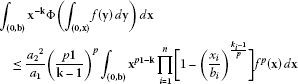(1.10)

holds for all non-negative integrable functions $f:\left(\mathbf{0},\mathbf{b}\right)\to \mathbb{R}$. The same inequality holds also if $p<0$, $\mathbf{k}<\mathbf{1}$, and f is an a.e. positive function. In the same paper, the so-called dual inequality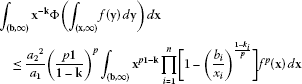(1.11)

was obtained, which holds for $p>1$, $\mathbf{b}\in \left[\mathbf{0},\mathrm{\infty }\right)$, $\mathbf{k}<\mathbf{1}$, and all non-negative integrable functions $f:\left(\mathbf{b},\mathrm{\infty }\right)\to \mathbb{R}$, as well as for $p<0$, $\mathbf{k}>\mathbf{1}$, and all a.e. positive integrable functions f on $\left(\mathbf{b},\mathrm{\infty }\right)$.

Motivated by the idea from , in this paper we generalize the modular Hardy-type inequality (1.2) to the class of arbitrary non-negative modular functions Φ equivalent to a non-negative convex function, that is, such that ${a}_{1}\mathrm{\Psi }\le \mathrm{\Phi }\le {a}_{2}\mathrm{\Psi }$ holds for some real constants ${a}_{2}>{a}_{1}\ge 0$ and a non-negative convex function Ψ. Applying the result obtained to some particular one-dimensional settings, we get new generalizations of the classical inequalities (1.4)-(1.7). Moreover, our result provides a new generalization of Godunova’s inequality (1.8) and improves inequalities (1.10) and (1.11) by relaxing the conditions on the function Φ and by replacing the constant $\frac{{a}_{2}^{2}}{{a}_{1}}$ with the smaller constant ${a}_{2}$. Finally, we show that a similar idea can be applied to the function Φ bounded with a superquadratic function Ψ in the same way. Such an approach enables us to get a new generalization of the refined Hardy-type inequality from .

Conventions Throughout this paper, all functions are assumed to be measurable and expressions of the form $0\cdot \mathrm{\infty }$, $\frac{0}{0}$, $\frac{\mathrm{\infty }}{\mathrm{\infty }}$, and $\frac{a}{\mathrm{\infty }}$, where $a\in \mathbb{R}$, are taken to be equal to zero. For a real parameter $0\ne p\ne 1$, by ${p}^{\prime }$ we denote its conjugate exponent ${p}^{\prime }=\frac{p}{p-1}$, that is, $\frac{1}{p}+\frac{1}{{p}^{\prime }}=1$. In addition, by a weight function (shortly: a weight), we mean a non-negative measurable function on the actual set, while an interval $I\subseteq \mathbb{R}$ stands for any convex subset of . As usual, logx is the natural logarithm of $x\in {\mathbb{R}}_{+}$, $B\left(\cdot ,\cdot \right)$ is the usual beta function, while $B\left(\cdot ;\cdot ,\cdot \right)$ denotes the incomplete beta function defined by

$B\left(x;a,b\right)={\int }_{0}^{x}{t}^{a-1}{\left(1-t\right)}^{b-1}\phantom{\rule{0.2em}{0ex}}dt,\phantom{\rule{1em}{0ex}}x\in \left[0,1\right],a,b>0.$

## 2 General Hardy-Knopp-type inequalities

Our first result is a generalization of inequality (1.2) to an arbitrary modular function $\mathrm{\Phi }:I\subseteq \mathbb{R}\to \mathbb{R}$ such that

${a}_{1}\mathrm{\Psi }\left(x\right)\le \mathrm{\Phi }\left(x\right)\le {a}_{2}\mathrm{\Psi }\left(x\right),\phantom{\rule{1em}{0ex}}x\in I,$
(2.1)

holds for some real constants $0<{a}_{1}\le {a}_{2}<\mathrm{\infty }$ and a non-negative convex function Ψ on I. For example, a whole class of such non-convex functions is given by $\mathrm{\Phi }\left(x\right)=\left[{a}_{1}+\left({a}_{2}-{a}_{1}\right)|sinx|\right]\mathrm{\Psi }\left(x\right)$, $x\in I$. Another interesting non-convex function Φ is given on ${\mathbb{R}}_{+}$ by

$\mathrm{\Phi }\left(x\right)=\left\{\begin{array}{cc}{x}^{p},\hfill & x\in \left(0,1\right),\hfill \\ {2}^{n},\hfill & x\in \left[{2}^{\frac{n-1}{p}},{2}^{\frac{n}{p}}\right),n\in \mathbb{N}.\hfill \end{array}$

It is equivalent to the power function $\mathrm{\Psi }\left(x\right)={x}^{p}$, where $p\in \left[1,\mathrm{\infty }\right)$, since it fulfills ${x}^{p}\le \mathrm{\Phi }\left(x\right)\le 2{x}^{p}$, $x\in {\mathbb{R}}_{+}$.

Now, we state and prove the central theorem in this section.

Theorem 2.1 Let $t\in \left[1,\mathrm{\infty }\right)$, $\left({\mathrm{\Omega }}_{1},{\mathrm{\Sigma }}_{1},{\mu }_{1}\right)$ and $\left({\mathrm{\Omega }}_{2},{\mathrm{\Sigma }}_{2},{\mu }_{2}\right)$ be measure spaces with positive σ-finite measures, u be a weight function on ${\mathrm{\Omega }}_{1}$, and k be a non-negative measurable function on ${\mathrm{\Omega }}_{1}×{\mathrm{\Omega }}_{2}$. Suppose that $K:{\mathrm{\Omega }}_{1}\to \mathbb{R}$ is as in (1.1), that the function $x↦u\left(x\right){\left(\frac{k\left(x,y\right)}{K\left(x\right)}\right)}^{t}$ is integrable on ${\mathrm{\Omega }}_{1}$ for each fixed $y\in {\mathrm{\Omega }}_{2}$, and that the weight function v is defined by

$v\left(y\right)={\left({\int }_{{\mathrm{\Omega }}_{1}}u\left(x\right){\left(\frac{k\left(x,y\right)}{K\left(x\right)}\right)}^{t}\phantom{\rule{0.2em}{0ex}}d{\mu }_{1}\left(x\right)\right)}^{\frac{1}{t}}<\mathrm{\infty },\phantom{\rule{1em}{0ex}}y\in {\mathrm{\Omega }}_{2}.$

If Φ and Ψ are non-negative functions on an interval $I\subseteq \mathbb{R}$, such that Ψ is convex and (2.1) holds for some real constants $0<{a}_{1}\le {a}_{2}<\mathrm{\infty }$, then the inequality

$\begin{array}{rcl}{\int }_{{\mathrm{\Omega }}_{1}}u\left(x\right){\mathrm{\Phi }}^{t}\left({A}_{k}f\left(x\right)\right)\phantom{\rule{0.2em}{0ex}}d{\mu }_{1}\left(x\right)& \le & {a}_{2}^{t}{\left({\int }_{{\mathrm{\Omega }}_{2}}v\left(y\right)\mathrm{\Psi }\left(f\left(y\right)\right)\phantom{\rule{0.2em}{0ex}}d{\mu }_{2}\left(y\right)\right)}^{t}\\ \le & {\left(\frac{{a}_{2}}{{a}_{1}}\right)}^{t}{\left({\int }_{{\mathrm{\Omega }}_{2}}v\left(y\right)\mathrm{\Phi }\left(f\left(y\right)\right)\phantom{\rule{0.2em}{0ex}}d{\mu }_{2}\left(y\right)\right)}^{t}\end{array}$
(2.2)

holds for all measurable functions $f:{\mathrm{\Omega }}_{2}\to \mathbb{R}$ with values in I and for ${A}_{k}f$ defined on ${\mathrm{\Omega }}_{1}$ by (1.3).

Proof Observe that ${A}_{k}f\left(x\right)\in I$, $x\in {\mathrm{\Omega }}_{1}$. Applying (2.1), Jensen’s and Minkowski’s inequalities as well as monotonicity of the power function $\alpha ↦{\alpha }^{t}$ on ${\mathbb{R}}_{+}$, we get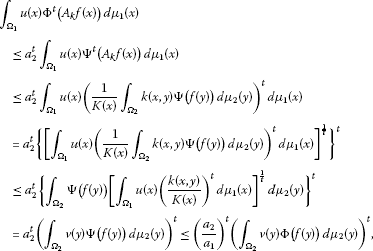so the proof is completed. □

Remark 2.1 Notice that the inequality

${\left({\int }_{{\mathrm{\Omega }}_{1}}u\left(x\right){\mathrm{\Phi }}^{t}\left({A}_{k}f\left(x\right)\right)\phantom{\rule{0.2em}{0ex}}d{\mu }_{1}\left(x\right)\right)}^{\frac{1}{t}}\le {\int }_{{\mathrm{\Omega }}_{2}}v\left(y\right)\mathrm{\Psi }\left(f\left(y\right)\right)\phantom{\rule{0.2em}{0ex}}d{\mu }_{2}\left(y\right)$

holds even if a non-negative function Φ is bounded with a convex function Ψ only from above, that is, if $0\le \mathrm{\Phi }\left(x\right)\le \mathrm{\Psi }\left(x\right)$, for a.e. $x\in I$. Therefore,

${\left({\int }_{{\mathrm{\Omega }}_{1}}u\left(x\right){\mathrm{\Phi }}^{t}\left({A}_{k}f\left(x\right)\right)\phantom{\rule{0.2em}{0ex}}d{\mu }_{1}\left(x\right)\right)}^{\frac{1}{t}}\le \underset{\mathrm{\Psi }\in \mathcal{C}}{inf}{\int }_{{\mathrm{\Omega }}_{2}}v\left(y\right)\mathrm{\Psi }\left(f\left(y\right)\right)\phantom{\rule{0.2em}{0ex}}d{\mu }_{2}\left(y\right),$

where $\mathcal{C}$ denotes the class of all convex functions Ψ on I such that $\mathrm{\Psi }\left(x\right)\ge \mathrm{\Phi }\left(x\right)$ for a.e. $x\in I$.

Remark 2.2 Rewriting (2.2) with $t=\frac{q}{p}\ge 1$, that is, with $0 or $-\mathrm{\infty }, we obtain

$\begin{array}{rcl}{\int }_{{\mathrm{\Omega }}_{1}}u\left(x\right){\mathrm{\Phi }}^{\frac{q}{p}}\left({A}_{k}f\left(x\right)\right)\phantom{\rule{0.2em}{0ex}}d{\mu }_{1}\left(x\right)& \le & {a}_{2}^{\frac{q}{p}}{\left({\int }_{{\mathrm{\Omega }}_{2}}v\left(y\right)\mathrm{\Psi }\left(f\left(y\right)\right)\phantom{\rule{0.2em}{0ex}}d{\mu }_{2}\left(y\right)\right)}^{\frac{q}{p}}\\ \le & {\left(\frac{{a}_{2}}{{a}_{1}}\right)}^{\frac{q}{p}}{\left({\int }_{{\mathrm{\Omega }}_{2}}v\left(y\right)\mathrm{\Phi }\left(f\left(y\right)\right)\phantom{\rule{0.2em}{0ex}}d{\mu }_{2}\left(y\right)\right)}^{\frac{q}{p}}.\end{array}$
(2.3)

Notice that for $p\ge 1$ or $p<0$ (in the latter case Φ and Ψ have to be positive), the function ${\mathrm{\Psi }}^{p}$ is convex as well. Hence, by replacing Φ with ${\mathrm{\Phi }}^{p}$ and considering that

${a}_{1}^{p}{\mathrm{\Psi }}^{p}\left(x\right)\le {\mathrm{\Phi }}^{p}\left(x\right)\le {a}_{2}^{p}{\mathrm{\Psi }}^{p}\left(x\right),\phantom{\rule{1em}{0ex}}x\in I,$

relation (2.3) becomes

${\parallel \mathrm{\Phi }\left({A}_{k}f\right)\parallel }_{{L}_{u}^{q}\left({\mathrm{\Omega }}_{1},{\mu }_{1}\right)}^{q}\le {a}_{2}^{q}{\parallel \mathrm{\Psi }f\parallel }_{{L}_{v}^{p}\left({\mathrm{\Omega }}_{2},{\mu }_{2}\right)}^{q}\le {\left(\frac{{a}_{2}}{{a}_{1}}\right)}^{q}{\parallel \mathrm{\Phi }f\parallel }_{{L}_{v}^{p}\left({\mathrm{\Omega }}_{2},{\mu }_{2}\right)}^{q}.$

Especially, for $1 and $\mathrm{\Psi }\left(x\right)=x$, we get

${\parallel \mathrm{\Phi }\left({A}_{k}f\right)\parallel }_{{L}_{u}^{q}\left({\mathrm{\Omega }}_{1},{\mu }_{1}\right)}^{q}\le {a}_{2}^{q}{\parallel f\parallel }_{{L}_{v}^{p}\left({\mathrm{\Omega }}_{2},{\mu }_{2}\right)}^{q}\le {\left(\frac{{a}_{2}}{{a}_{1}}\right)}^{q}{\parallel \mathrm{\Phi }f\parallel }_{{L}_{v}^{p}\left({\mathrm{\Omega }}_{2},{\mu }_{2}\right)}^{q}.$

Remark 2.3 For ${a}_{1}={a}_{2}=1$, inequality (2.2) reduces to (1.2), so Theorem 2.1 can be regarded as a generalization of the corresponding result from . In that case, the function Φ has to be convex.

The following two sections are dedicated to some applications and analogues of Theorem 2.1. Namely, by choosing some standard measure spaces, kernels, and weight functions, we get generalizations of one-dimensional and multidimensional Hardy-Knopp-type inequalities from the papers [1, 4, 79], and .

## 3 Generalized one-dimensional Hardy-Knopp-type inequalities

In this section, we consider the standard one-dimensional setting with intervals in and the Lebesgue measure. First, let $0 and

${S}_{1}=\left\{\left(x,y\right)\in {\mathbb{R}}^{2}:0
(3.1)

Applying Theorem 2.1 to ${\mathrm{\Omega }}_{1}={\mathrm{\Omega }}_{2}=\left(0,b\right)$, $d{\mu }_{1}\left(x\right)=dx$, $d{\mu }_{2}\left(y\right)=dy$, and to $k{\chi }_{{S}_{1}}$, $\frac{u\left(x\right)}{x}$, $\frac{w\left(y\right)}{y}$ instead of k, $u\left(x\right)$, $v\left(y\right)$, we get the following corollary.

Corollary 3.1 Let $0 and $k:\left(0,b\right)×\left(0,b\right)\to \mathbb{R}$, $u:\left(0,b\right)\to \mathbb{R}$ be non-negative measurable functions such that

$K\left(x\right)={\int }_{0}^{x}k\left(x,y\right)\phantom{\rule{0.2em}{0ex}}dy>0,\phantom{\rule{1em}{0ex}}x\in \left(0,b\right),$
(3.2)

and let

$w\left(y\right)=y{\left({\int }_{y}^{b}u\left(x\right){\left(\frac{k\left(x,y\right)}{K\left(x\right)}\right)}^{\frac{q}{p}}\frac{dx}{x}\right)}^{\frac{p}{q}}<\mathrm{\infty },\phantom{\rule{1em}{0ex}}y\in \left(0,b\right).$

If $0 or $-\mathrm{\infty }, Ψ is a non-negative convex function on an interval $I\subseteq \mathbb{R}$, and $\mathrm{\Phi }:I\to \mathbb{R}$ fulfills (2.1) for some positive real constants ${a}_{1}\le {a}_{2}$, then the inequalities

$\begin{array}{rcl}{\int }_{0}^{b}u\left(x\right){\mathrm{\Phi }}^{\frac{q}{p}}\left({A}_{k}f\left(x\right)\right)\frac{dx}{x}& \le & {a}_{2}^{\frac{q}{p}}{\left({\int }_{0}^{b}w\left(y\right)\mathrm{\Psi }\left(f\left(y\right)\right)\frac{dy}{y}\right)}^{\frac{q}{p}}\\ \le & {\left(\frac{{a}_{2}}{{a}_{1}}\right)}^{\frac{q}{p}}{\left({\int }_{0}^{b}w\left(y\right)\mathrm{\Phi }\left(f\left(y\right)\right)\frac{dy}{y}\right)}^{\frac{q}{p}}\end{array}$
(3.3)

hold for all measurable functions $f:\left(0,b\right)\to \mathbb{R}$ with values in I and for ${A}_{k}f$ defined by

${A}_{k}f\left(x\right)=\frac{1}{K\left(x\right)}{\int }_{0}^{x}k\left(x,y\right)f\left(y\right)\phantom{\rule{0.2em}{0ex}}dy,\phantom{\rule{1em}{0ex}}x\in \left(0,b\right).$
(3.4)

On the other hand, for $0\le b<\mathrm{\infty }$,

${S}_{2}=\left\{\left(x,y\right)\in {\mathbb{R}}^{2}:b
(3.5)

${\mathrm{\Omega }}_{1}={\mathrm{\Omega }}_{2}=\left(b,\mathrm{\infty }\right)$, $d{\mu }_{1}\left(x\right)=dx$, $d{\mu }_{2}\left(y\right)=dy$, and for k, $u\left(x\right)$, and $v\left(y\right)$ respectively replaced with $k{\chi }_{{S}_{2}}$, $\frac{u\left(x\right)}{x}$, and $\frac{w\left(y\right)}{y}$, Theorem 2.1 provides the results dual to Corollary 3.1.

Corollary 3.2 For $0\le b<\mathrm{\infty }$, let $k:\left(b,\mathrm{\infty }\right)×\left(b,\mathrm{\infty }\right)\to \mathbb{R}$ and $u:\left(b,\mathrm{\infty }\right)\to \mathbb{R}$ be non-negative measurable functions satisfying

$\stackrel{˜}{K}\left(x\right)={\int }_{x}^{\mathrm{\infty }}k\left(x,y\right)\phantom{\rule{0.2em}{0ex}}dy>0,\phantom{\rule{1em}{0ex}}x\in \left(b,\mathrm{\infty }\right),$
(3.6)

and

$\stackrel{˜}{w}\left(y\right)=y{\left({\int }_{b}^{y}u\left(x\right){\left(\frac{k\left(x,y\right)}{\stackrel{˜}{K}\left(x\right)}\right)}^{\frac{q}{p}}\frac{dx}{x}\right)}^{\frac{p}{q}}<\mathrm{\infty },\phantom{\rule{1em}{0ex}}y\in \left(b,\mathrm{\infty }\right).$

If $0 or $-\mathrm{\infty }, Ψ is a non-negative convex function on an interval $I\subseteq \mathbb{R}$ and $\mathrm{\Phi }:I\to \mathbb{R}$ satisfies (2.1), then the inequalities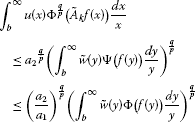(3.7)

hold for all measurable functions $f:\left(b,\mathrm{\infty }\right)\to \mathbb{R}$ with values in I and for ${\stackrel{˜}{A}}_{k}f$ defined as

${\stackrel{˜}{A}}_{k}f\left(x\right)=\frac{1}{\stackrel{˜}{K}\left(x\right)}{\int }_{x}^{\mathrm{\infty }}k\left(x,y\right)f\left(y\right)\phantom{\rule{0.2em}{0ex}}dy,\phantom{\rule{1em}{0ex}}x\in \left(b,\mathrm{\infty }\right).$

Remark 3.1 By setting $p=q\in \mathbb{R}\setminus \left\{0\right\}$ in Corollary 3.1 and Corollary 3.2, we obtain a generalization of [, Theorem 3.1] and [, Theorem 4.3]. Observe that the functions Φ and Ψ need not be non-negative in that case.

As a consequence of Corollary 3.1, we get an inequality related to the so-called Riemann-Liouville operator.

Example 3.1 Let b, p, and q be as in Corollary 3.1, the set ${S}_{1}$ be defined by (3.1), and let $k:\left(0,b\right)×\left(0,b\right)\to \mathbb{R}$ and $u:\left(0,b\right)\to \mathbb{R}$ be defined by $k\left(x,y\right)=\frac{\gamma }{{x}^{\gamma }}{\left(x-y\right)}^{\gamma -1}{\chi }_{{S}_{1}}\left(x,y\right)$ and $u\left(x\right)\equiv 1$, where $0\le 1-\frac{p}{q}<\gamma <\mathrm{\infty }$. Under the conditions of Corollary 3.1, we have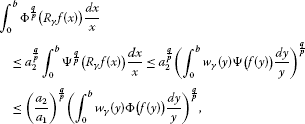(3.8)

where ${R}_{\gamma }$ is Riemann-Liouville’s operator given by

${R}_{\gamma }f\left(x\right)=\frac{\gamma }{{x}^{\gamma }}{\int }_{0}^{x}{\left(x-y\right)}^{\gamma -1}f\left(y\right)\phantom{\rule{0.2em}{0ex}}dy,\phantom{\rule{1em}{0ex}}x\in \left(0,b\right),$
(3.9)

while for $x,y\in \left(0,b\right)$, we define

${w}_{\gamma }\left(y\right)=\gamma {\left({\int }_{0}^{1-\frac{y}{b}}{t}^{\left(\gamma -1\right)\frac{q}{p}}{\left(1-t\right)}^{\frac{q}{p}-1}\phantom{\rule{0.2em}{0ex}}dt\right)}^{\frac{p}{q}}=\gamma {B}^{\frac{p}{q}}\left(1-\frac{y}{b};\left(\gamma -1\right)\frac{q}{p}+1,\frac{q}{p}\right).$

As usual, $B\left(\cdot ;\cdot ,\cdot \right)$ denotes the incomplete beta function defined in the introduction. Rewriting the second line of (3.8) with $\mathrm{\Psi }\left(x\right)={x}^{p}$, $k\in \mathbb{R}$ such that $\frac{k-1}{p}>0$, and with ${b}^{\frac{k-1}{p}}$ and $f\left({y}^{\frac{p}{k-1}}\right){y}^{\frac{p}{k-1}-1}$ instead of b and $f\left(y\right)$, after a sequence of suitable variable changes, we obtain the strengthened Hardy inequality

${\int }_{0}^{b}{x}^{\frac{q}{p}\left(1-k\right)-1}{\left(Rf\left(x\right)\right)}^{q}\phantom{\rule{0.2em}{0ex}}dx\le \gamma {\left(\frac{p}{\gamma \left(k-1\right)}\right)}^{q+1-\frac{q}{p}}{\left({\int }_{0}^{b}{w}_{\gamma ,k}\left(y\right){f}^{p}\left(y\right)\phantom{\rule{0.2em}{0ex}}dy\right)}^{\frac{q}{p}}$
(3.10)

for non-negative functions f on $\left(0,b\right)$ (positive, if $p<0$), where

${w}_{\gamma ,k}\left(y\right)={B}^{\frac{p}{q}}\left(1-{\left(\frac{y}{b}\right)}^{\frac{k-1}{p}};\left(\gamma -1\right)\frac{q}{p}+1,\frac{q}{p}\right){y}^{p-k},\phantom{\rule{1em}{0ex}}y\in \left(0,b\right),$

and

$Rf\left(x\right)={\int }_{0}^{x}{\left[1-{\left(\frac{y}{x}\right)}^{\frac{k-1}{p}}\right]}^{\gamma -1}f\left(y\right)\phantom{\rule{0.2em}{0ex}}dy,\phantom{\rule{1em}{0ex}}x\in \left(0,b\right)$

(see  for more details). If the function Φ is such that (1.9) holds, then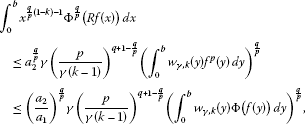(3.11)

so we obtained a new generalization of (3.10). Notice that for $b=\mathrm{\infty }$, $k=q=p>1$, $\gamma =1$, and $\mathrm{\Phi }\left(x\right)={x}^{p}$, $x\in {\mathbb{R}}_{+}$, inequalities (3.11) reduce to the classical Hardy inequality (1.4).

On the other hand, rewriting the second line of (3.8) with $\mathrm{\Psi }\left(x\right)={e}^{x}$ and $\gamma =1$, as well as with $y↦log\left(yf\left(y\right)\right)$ instead of a positive function $f:\left(0,b\right)\to \mathbb{R}$, we get the strengthened Pólya-Knopp inequality

${\int }_{0}^{b}{x}^{\frac{q}{p}-1}{\left(Gf\left(x\right)\right)}^{\frac{q}{p}}\phantom{\rule{0.2em}{0ex}}dx\le \frac{p}{q}{e}^{\frac{q}{p}}{\left({\int }_{0}^{b}{\left[1-{\left(\frac{y}{b}\right)}^{\frac{q}{p}}\right]}^{\frac{p}{q}}f\left(y\right)\phantom{\rule{0.2em}{0ex}}dy\right)}^{\frac{q}{p}},$
(3.12)

obtained in , where

$Gf\left(x\right)=exp\left(\frac{1}{x}{\int }_{0}^{x}logf\left(y\right)\phantom{\rule{0.2em}{0ex}}dy\right),\phantom{\rule{1em}{0ex}}x\in \left(0,b\right).$

Hence, for the function $\mathrm{\Phi }:{\mathbb{R}}_{+}\to \mathbb{R}$ satisfying ${a}_{1}x\le \mathrm{\Phi }\left(x\right)\le {a}_{2}x$ for a.e. $x\in \mathbb{R}$, where $0<{a}_{1}\le {a}_{2}<\mathrm{\infty }$, we get the following generalization of (3.12):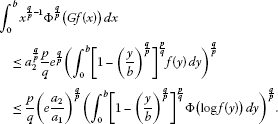(3.13)

Observe that inequality (1.7) follows from (3.13) by taking $b=\mathrm{\infty }$, $p=q$ and $\mathrm{\Phi }\left(x\right)=x$, $x\in {\mathbb{R}}_{+}$.

In the sequel, we state and prove inequalities dual to (3.8)-(3.13), related to the so-called Weyl operator.

Example 3.2 Suppose $0\le b<\mathrm{\infty }$ and ${S}_{2}$ is defined by (3.5). Define the kernel $k:\left(b,\mathrm{\infty }\right)×\left(b,\mathrm{\infty }\right)\to \mathbb{R}$ and the weight function $u:\left(b,\mathrm{\infty }\right)\to \mathbb{R}$ as $k\left(x,y\right)=\gamma \frac{x}{{y}^{\gamma +1}}{\left(y-x\right)}^{\gamma -1}{\chi }_{{S}_{2}}\left(x,y\right)$ and $u\left(x\right)\equiv 1$. For $\gamma ,p,q\in \mathbb{R}$, $\frac{q}{p}\ge 1$, $\gamma >1-\frac{p}{q}$, a non-negative function Φ on an interval $I\subseteq \mathbb{R}$, a convex function $\mathrm{\Psi }:I\to \mathbb{R}$ fulfilling (2.1), and a function $f:\left(b,\mathrm{\infty }\right)\to \mathbb{R}$ with values in I, from Corollary 3.2 we get the inequalities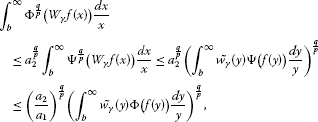(3.14)

where ${W}_{\gamma }$ denotes the Weyl operator ${W}_{\gamma }$ given by

${W}_{\gamma }f\left(x\right)=\gamma x{\int }_{x}^{\mathrm{\infty }}{\left(y-x\right)}^{\gamma -1}f\left(y\right)\frac{dy}{{y}^{\gamma +1}},\phantom{\rule{1em}{0ex}}x\in \left(b,\mathrm{\infty }\right),$
(3.15)

and for $x,y\in \left(b,\mathrm{\infty }\right)$, we define ${\stackrel{˜}{w}}_{\gamma }\left(y\right)=\gamma {B}^{\frac{p}{q}}\left(1-\frac{b}{y};\left(\gamma -1\right)\frac{q}{p}+1,\frac{q}{p}\right)$.

As in Example 3.1, to get a new dual Hardy inequality, we rewrite (3.14) with $\mathrm{\Psi }\left(x\right)={x}^{p}$. More precisely, let $k\in \mathbb{R}$ be such that $\frac{p}{1-k}>0$,

${\stackrel{˜}{w}}_{\gamma ,k}\left(y\right)={B}^{\frac{p}{q}}\left(1-{\left(\frac{b}{y}\right)}^{\frac{1-k}{p}};\left(\gamma -1\right)\frac{q}{p}+1,\frac{q}{p}\right){y}^{p-k},\phantom{\rule{1em}{0ex}}y\in \left(b,\mathrm{\infty }\right),$

f be a non-negative function on $\left(b,\mathrm{\infty }\right)$ (positive, if $p<0$), and

$Wf\left(x\right)={\int }_{x}^{\mathrm{\infty }}{\left[1-{\left(\frac{x}{y}\right)}^{\frac{1-k}{p}}\right]}^{\gamma -1}f\left(y\right)\phantom{\rule{0.2em}{0ex}}dy,\phantom{\rule{1em}{0ex}}x\in \left(b,\mathrm{\infty }\right).$

Substituting ${b}^{\frac{1-k}{p}}$ and $f\left({y}^{\frac{p}{1-k}}\right){y}^{\frac{p}{1-k}+1}$ respectively for b and $f\left(y\right)$ in the inequality from the second line of (3.14), after some computations and using the condition (1.9), as in , we obtain the inequalities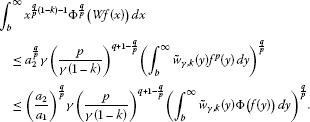(3.16)

For $b=0$, $k=q=p>1$, $\gamma =1$, and $\mathrm{\Phi }\left(x\right)={x}^{p}$, $x\in {\mathbb{R}}_{+}$ relation (3.16) reduces to the so-called classical dual Hardy inequality.

Finally, for $\gamma =1$, $\mathrm{\Psi }\left(x\right)=x$, and $y↦log\left(yf\left(y\right)\right)$ instead of a positive function $f:\left(b,\mathrm{\infty }\right)\to \mathbb{R}$, inequality (3.14) becomes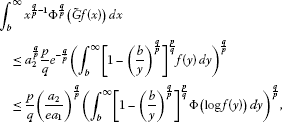(3.17)

where

$\stackrel{˜}{G}f\left(x\right)=exp\left(x{\int }_{x}^{\mathrm{\infty }}logf\left(y\right)\frac{dy}{{y}^{2}}\right),\phantom{\rule{1em}{0ex}}y\in \left(b,\mathrm{\infty }\right).$

Since for $b=0$, $p=q$, and $\mathrm{\Phi }\left(x\right)=x$, $x\in {\mathbb{R}}_{+}$, relation (3.17) reduces to the so-called classical dual Pólya-Knopp inequality, our result can be regarded as its generalization.

Remark 3.2 It is important to notice that due to variable changes applied, none of the inequalities from Example 3.1 and Example 3.2 can be derived directly from Theorem 2.1.

Our analysis continues by considering ${\mathrm{\Omega }}_{1}={\mathrm{\Omega }}_{2}={\mathbb{R}}_{+}$. We still assume that $d{\mu }_{1}\left(x\right)=dx$ and $d{\mu }_{2}\left(y\right)=dy$. In the following example, we apply Theorem 2.1 to provide a new generalization of the classical Hardy-Hilbert inequality (1.5).

Example 3.3 Let $p,q,s\in \mathbb{R}$ be such that $\frac{q}{p}\ge 1$ and $\frac{s-2}{p},\frac{s-2}{{p}^{\prime }}>-1$, and let $\alpha \in \left(-\frac{q}{p}\left(\frac{s-2}{{p}^{\prime }}+1\right),\frac{q}{p}\left(\frac{s-2}{p}+1\right)\right)$. Let $k:{\mathbb{R}}_{+}^{2}\to \mathbb{R}$ and $u:{\mathbb{R}}_{+}\to \mathbb{R}$ be respectively defined by $k\left(x,y\right)={\left(\frac{y}{x}\right)}^{\frac{s-2}{p}}{\left(x+y\right)}^{-s}$ and $u\left(x\right)={x}^{\alpha -1}$. Applying Theorem 2.1 with $\mathrm{\Psi }\left(x\right)={x}^{p}$ and with $f\left(y\right){y}^{\frac{2-s}{p}}$ instead of $f\left(y\right)$, as in , we get the inequalities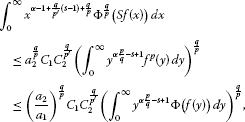(3.18)

where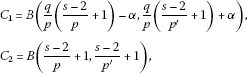and Sf denotes the generalized Stieltjes transform of a non-negative function f on ${\mathbb{R}}_{+}$,

$Sf\left(x\right)={\int }_{0}^{\mathrm{\infty }}\frac{f\left(y\right)}{{\left(x+y\right)}^{s}}\phantom{\rule{0.2em}{0ex}}dy,\phantom{\rule{1em}{0ex}}x\in {\mathbb{R}}_{+}$

(see  and  for further information). In particular, for $p=q>1$, $\alpha =0$, and $s=1$, we have ${C}_{1}={C}_{2}=B\left(\frac{1}{p},\frac{1}{{p}^{\prime }}\right)=\frac{\pi }{sin\frac{\pi }{p}}$, so (3.18) provides a new generalization of the classical Hardy-Hilbert inequality (1.5).

Similarly, in the next example we generalize the classical Hardy-Littlewood-Pólya inequality (1.6).

Example 3.4 Let the parameters p, q, s, α and the functions u and f be as in Example 3.3, and let $k:{\mathbb{R}}_{+}^{2}\to \mathbb{R}$ be defined by $k\left(x,y\right)={\left(\frac{y}{x}\right)}^{\frac{s-2}{p}}max{\left\{x,y\right\}}^{-s}$. For a non-negative function Φ such that (1.9) holds, and for $f\left(y\right){y}^{\frac{2-s}{p}}$ instead of $f\left(y\right)$, from Theorem 2.1 we get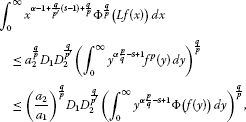(3.19)

where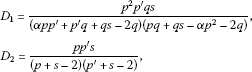and

$Lf\left(x\right)={\int }_{0}^{\mathrm{\infty }}\frac{f\left(y\right)}{max{\left\{x,y\right\}}^{s}}\phantom{\rule{0.2em}{0ex}}dy,\phantom{\rule{1em}{0ex}}x\in {\mathbb{R}}_{+}$

(see  for more details). For $p=q>1$, $\alpha =0$, and $s=1$, we have ${D}_{1}={D}_{2}=p{p}^{\prime }$, so it is not hard to see that our result generalizes the classical Hardy-Littlewood-Pólya inequality (1.6).

We complete this section with another Hardy-Hilbert-type inequality, making use of the well-known reflection formula for the Digamma function ψ,

${\int }_{0}^{\mathrm{\infty }}\frac{logt}{t-1}{t}^{-\alpha }\phantom{\rule{0.2em}{0ex}}dt={\psi }^{\prime }\left(1-\alpha \right)+{\psi }^{\prime }\left(\alpha \right)=\frac{{\pi }^{2}}{{sin}^{2}\pi \alpha },\phantom{\rule{1em}{0ex}}\alpha \in \left(0,1\right),$

and of the fact that

$Z\left(a,b\right)={\int }_{0}^{\mathrm{\infty }}{t}^{b}{e}^{-at}{\left(1-{e}^{-t}\right)}^{b}\phantom{\rule{0.2em}{0ex}}dt<\mathrm{\infty },\phantom{\rule{1em}{0ex}}a\in {\mathbb{R}}_{+},b\ge 1.$

More precisely, $Z\left(a,b\right)=\mathrm{\Gamma }\left(b+1\right){\varphi }_{b}^{\ast }\left(1,b+1,a\right)$, where ${\varphi }_{\mu }^{\ast }$ is the so-called unified Riemann-Zeta function,

${\varphi }_{\mu }^{\ast }\left(z,s,a\right)=\frac{1}{\mathrm{\Gamma }\left(s\right)}{\int }_{0}^{\mathrm{\infty }}{t}^{s-1}{e}^{-at}{\left(1-z{e}^{-t}\right)}^{-\mu }\phantom{\rule{0.2em}{0ex}}dt,$

where $\mu \ge 1$, $Rea>0$ and either $|z|\le 1$, $z\ne 1$ and $Res>0$ or $z=1$ and $Res>\mu$ (for more information regarding the unified Riemann-Zeta function, see, e.g., ).

Example 3.5 Suppose that $\alpha \in \left(0,1\right)$ and $p,q,\beta \in \mathbb{R}$ are such that $\frac{q}{p}\ge 1$ and $\alpha \frac{q}{p}+\beta \in \left(-1,\frac{q}{p}-1\right)$. Define the kernel $k:{\mathbb{R}}_{+}^{2}\to \mathbb{R}$ by $k\left(x,y\right)=\frac{logy-logx}{y-x}{\left(\frac{x}{y}\right)}^{\alpha }$ and the weight function $u:{\mathbb{R}}_{+}\to \mathbb{R}$ by $u\left(x\right)={x}^{\beta }$. As in previous two examples, applying Theorem 2.1 to $\mathrm{\Psi }\left(x\right)={x}^{p}$ and to $f\left(y\right){y}^{\alpha }$ instead of $f\left(y\right)$, we get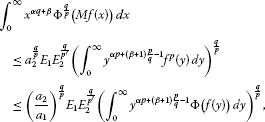(3.20)

where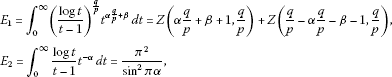and

$Mf\left(x\right)={\int }_{0}^{\mathrm{\infty }}\frac{logy-logx}{y-x}f\left(y\right)\phantom{\rule{0.2em}{0ex}}dy,\phantom{\rule{1em}{0ex}}x\in {\mathbb{R}}_{+}.$

Observe that for $\mathrm{\Phi }\left(x\right)={x}^{p}$ relation (3.20) reduces to a usual Hardy-Hilbert-type inequality, so our result can be seen as a generalization in that direction.

## 4 Generalized multidimensional Hardy-Knopp-type inequalities

In this section, we give a multidimensional result related to Godunova’s inequality (1.8). Namely, we improve and generalize inequalities (1.10) and (1.11) by considering an arbitrary function Φ, not necessarily convex, such that (2.1) holds.

Suppose that ${\mathrm{\Omega }}_{1}={\mathrm{\Omega }}_{2}={\mathbb{R}}_{+}^{n}$, $d{\mu }_{1}\left(\mathbf{x}\right)=d\mathbf{x}$, $d{\mu }_{2}\left(\mathbf{y}\right)=d\mathbf{y}$, and that the kernel $k:{\mathbb{R}}_{+}^{n}×{\mathbb{R}}_{+}^{n}\to \mathbb{R}$ is of the form $k\left(\mathbf{x},\mathbf{y}\right)=l\left(\frac{\mathbf{y}}{\mathbf{x}}\right)$, where $l:{\mathbb{R}}_{+}^{n}\to \mathbb{R}$ is a non-negative measurable function. Applying Theorem 2.1 to this setting and to $u\left(\mathbf{x}\right)$ and $v\left(\mathbf{y}\right)$, respectively replaced with $\frac{u\left(\mathbf{x}\right)}{{\mathbf{x}}^{\mathbf{1}}}$ and $\frac{w\left(\mathbf{y}\right)}{{\mathbf{y}}^{\mathbf{1}}}$, we get the following generalization of Godunova’s inequality (1.8) and a generalization of [, Theorem 6.1].

Theorem 4.1 Let $0 or $-\mathrm{\infty }. Let l and u be non-negative measurable functions on ${\mathbb{R}}_{+}^{n}$ such that $0 for all $\mathbf{x}\in {\mathbb{R}}_{+}^{n}$, and that the function $\mathbf{x}↦u\left(\mathbf{x}\right){\left(\frac{l\left(\frac{\mathbf{y}}{\mathbf{x}}\right)}{L\left(\mathbf{x}\right)}\right)}^{\frac{q}{p}}$ is integrable on ${\mathbb{R}}_{+}^{n}$ for each fixed $\mathbf{y}\in {\mathbb{R}}_{+}^{n}$. Let the function w be defined on ${\mathbb{R}}_{+}^{n}$ by

$w\left(\mathbf{y}\right)={\mathbf{y}}^{\mathbf{1}}{\left({\int }_{{\mathbb{R}}_{+}^{n}}u\left(\mathbf{x}\right){\left(\frac{l\left(\frac{\mathbf{y}}{\mathbf{x}}\right)}{L\left(\mathbf{x}\right)}\right)}^{\frac{q}{p}}\frac{d\mathbf{x}}{{\mathbf{x}}^{\mathbf{1}}}\right)}^{\frac{p}{q}}.$

If Ψ is a non-negative convex function on an interval $I\subseteq \mathbb{R}$ and $\mathrm{\Phi }:I\to \mathbb{R}$ is any function satisfying (2.1) for some real constants $0<{a}_{1}\le {a}_{2}<\mathrm{\infty }$, then the inequality

$\begin{array}{rcl}{\int }_{{\mathbb{R}}_{+}^{n}}u\left(\mathbf{x}\right){\mathrm{\Phi }}^{\frac{q}{p}}\left({A}_{l}f\left(\mathbf{x}\right)\right)\frac{d\mathbf{x}}{{\mathbf{x}}^{\mathbf{1}}}& \le & {a}_{2}^{\frac{q}{p}}{\left({\int }_{{\mathbb{R}}_{+}^{n}}w\left(\mathbf{y}\right)\mathrm{\Psi }\left(f\left(\mathbf{y}\right)\right)\frac{d\mathbf{y}}{{\mathbf{y}}^{\mathbf{1}}}\right)}^{\frac{q}{p}}\\ \le & {\left(\frac{{a}_{2}}{{a}_{1}}\right)}^{\frac{q}{p}}{\left({\int }_{{\mathbb{R}}_{+}^{n}}w\left(\mathbf{y}\right)\mathrm{\Phi }\left(f\left(\mathbf{y}\right)\right)\frac{d\mathbf{y}}{{\mathbf{y}}^{\mathbf{1}}}\right)}^{\frac{q}{p}}\end{array}$
(4.1)

holds for all measurable functions $f:{\mathbb{R}}_{+}^{n}\to \mathbb{R}$ with values in I and ${A}_{l}$ defined by

${A}_{l}f\left(\mathbf{x}\right)=\frac{1}{L\left(\mathbf{x}\right)}{\int }_{{\mathbb{R}}_{+}^{n}}l\left(\frac{\mathbf{y}}{\mathbf{x}}\right)f\left(\mathbf{y}\right)\phantom{\rule{0.2em}{0ex}}d\mathbf{y},\phantom{\rule{1em}{0ex}}\mathbf{x}\in {\mathbb{R}}_{+}^{n}.$

The above result can be reformulated with particular convex functions, for example, with power and exponential functions. This leads to multidimensional analogues of corollaries and examples from the previous section. Due to the lack of space, we only give a result regarding the n-dimensional Riemann-Liouville operator. The corresponding result for the n-dimensional Weyl operator, which provides a generalization and a refinement of (1.11), can be obtained by a similar method as in the one-dimensional case.

Following the idea that we have used to get inequality (3.11), we obtain the next result.

Example 4.1 Let $\gamma ,p,q\in \mathbb{R}$ be such that $0\le 1-\frac{p}{q}<\gamma$. Let $\mathbf{0}<\mathbf{b}\le \mathbf{\infty }$ and

${S}_{1}^{n}=\left\{\left(\mathbf{x},\mathbf{y}\right)\in {\mathbb{R}}^{n}×{\mathbb{R}}^{n}:\mathbf{0}<\mathbf{y}\le \mathbf{x}<\mathbf{b}\right\}.$
(4.2)

Specifying Theorem 4.1 for $u:{\mathbb{R}}_{+}^{n}\to \mathbb{R}$, $u\left(\mathbf{x}\right)={\chi }_{{S}_{1}^{n}}\left(\mathbf{x},\mathbf{x}\right)$, and $l:{\mathbb{R}}_{+}^{n}\to \mathbb{R}$, $l\left(\mathbf{x}\right)={\gamma }^{n}{\left(\mathbf{1}-\mathbf{x}\right)}^{\left(\gamma -1\right)\mathbf{1}}{\chi }_{\left(\mathbf{0},\mathbf{1}\right)}\left(\mathbf{x}\right)$, we get $L\left(\mathbf{x}\right)={\mathbf{x}}^{\mathbf{1}}$ and the inequalities

$\begin{array}{rcl}{\int }_{\left(\mathbf{0},\mathbf{b}\right)}{\mathrm{\Phi }}^{\frac{q}{p}}\left({R}_{\gamma ,n}f\left(\mathbf{x}\right)\right)\frac{d\mathbf{x}}{{\mathbf{x}}^{\mathbf{1}}}& \le & {a}_{2}^{\frac{q}{p}}{\int }_{\left(\mathbf{0},\mathbf{b}\right)}{\mathrm{\Psi }}^{\frac{q}{p}}\left({R}_{\gamma ,n}f\left(\mathbf{x}\right)\right)\frac{d\mathbf{x}}{{\mathbf{x}}^{\mathbf{1}}}\le {a}_{2}^{\frac{q}{p}}{\left({\int }_{\left(\mathbf{0},\mathbf{b}\right)}{w}_{\gamma ,n}\left(\mathbf{y}\right)\mathrm{\Psi }\left(f\left(\mathbf{y}\right)\right)\frac{d\mathbf{y}}{{\mathbf{y}}^{\mathbf{1}}}\right)}^{\frac{q}{p}}\\ \le & {\left(\frac{{a}_{2}}{{a}_{1}}\right)}^{\frac{q}{p}}{\left({\int }_{\left(\mathbf{0},\mathbf{b}\right)}{w}_{\gamma ,n}\left(\mathbf{y}\right)\mathrm{\Phi }\left(f\left(\mathbf{y}\right)\right)\frac{d\mathbf{y}}{{\mathbf{y}}^{\mathbf{1}}}\right)}^{\frac{q}{p}},\end{array}$
(4.3)

where

${R}_{\gamma ,n}f\left(\mathbf{x}\right)=\frac{{\gamma }^{n}}{{\mathbf{x}}^{\gamma \mathbf{1}}}{\int }_{\left(\mathbf{0},\mathbf{x}\right)}{\left(\mathbf{x}-\mathbf{y}\right)}^{\left(\gamma -1\right)\mathbf{1}}f\left(\mathbf{y}\right)\phantom{\rule{0.2em}{0ex}}d\mathbf{y},\phantom{\rule{1em}{0ex}}\mathbf{x}\in \left(\mathbf{0},\mathbf{b}\right),$
(4.4)

and

${w}_{\gamma ,n}\left(\mathbf{y}\right)={\gamma }^{n}\prod _{i=1}^{n}{B}^{\frac{p}{q}}\left(1-\frac{{y}_{i}}{{b}_{i}};\left(\gamma -1\right)\frac{q}{p}+1,\frac{q}{p}\right),\phantom{\rule{1em}{0ex}}\mathbf{y}\in \left(\mathbf{0},\mathbf{b}\right).$

Considering the inequality from the second line of (4.3) with $\mathrm{\Psi }\left(x\right)={x}^{p}$, $\mathbf{k}\in {\mathbb{R}}^{n}$ such that $\frac{\mathbf{k}-\mathbf{1}}{p\mathbf{1}}>\mathbf{0}$, and with $\mathbf{c}=\left({b}_{1}^{\frac{{k}_{1}-1}{p}},\dots ,{b}_{n}^{\frac{{k}_{n}-1}{p}}\right)$ and $f\left({y}_{1}^{\frac{p}{{k}_{1}-1}},\dots ,{y}_{n}^{\frac{p}{{k}_{n}-1}}\right){\mathbf{y}}^{\frac{p\mathbf{1}}{\mathbf{k}-\mathbf{1}}-\mathbf{1}}$, respectively instead of b and $f\left(\mathbf{y}\right)$, after a sequence of suitable variable changes, we obtain the inequality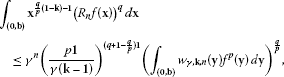(4.5)

where

${w}_{\gamma ,\mathbf{k},n}\left(\mathbf{y}\right)={\mathbf{y}}^{p\mathbf{1}-\mathbf{k}}\prod _{i=1}^{n}{B}^{\frac{p}{q}}\left(1-{\left(\frac{{y}_{i}}{{b}_{i}}\right)}^{\frac{{k}_{i}-1}{p}};\left(\gamma -1\right)\frac{q}{p}+1,\frac{q}{p}\right),\phantom{\rule{1em}{0ex}}\mathbf{y}\in \left(\mathbf{0},\mathbf{b}\right),$

and

${R}_{n}f\left(\mathbf{x}\right)={\int }_{\left(\mathbf{0},\mathbf{x}\right)}{\left\{\prod _{i=1}^{n}\left[1-{\left(\frac{{y}_{i}}{{x}_{i}}\right)}^{\frac{{k}_{i}-1}{p}}\right]\right\}}^{\gamma -1}f\left(\mathbf{y}\right)\phantom{\rule{0.2em}{0ex}}d\mathbf{y},\phantom{\rule{1em}{0ex}}\mathbf{x}\in \left(\mathbf{0},\mathbf{b}\right).$
(4.6)

Moreover, if the function Φ is such that (1.9) holds, then we get a new generalization of the strengthened Hardy inequality (4.5),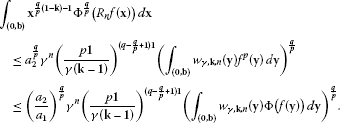(4.7)

Notice that (4.7) generalizes and refines inequality (1.10). Namely, for $p=q$ and $\gamma =1$, inequality (4.7) reduces to (1.10), only with a smaller constant $\frac{{a}_{2}}{{a}_{1}}\le \frac{{a}_{2}^{2}}{{a}_{1}}$ on its right-hand side (since $0<{a}_{1}\le {a}_{2}$).

## 5 General Hardy-type inequalities for superquadratic functions

To conclude the paper, we state and prove a weighted Hardy-type inequality involving general measure spaces, a non-negative kernel, and a function bounded with a superquadratic function. For reader’s convenience, we recall the notion and some basic properties of superquadratic functions (for more information, see ). A function $\phi :\left[0,\mathrm{\infty }\right)\to \mathbb{R}$ is called superquadratic provided that for each $x\ge 0$ there exists a constant ${C}_{x}\in \mathbb{R}$ such that

$\mathrm{\Psi }\left(y\right)-\mathrm{\Psi }\left(x\right)-\mathrm{\Psi }\left(|y-x|\right)\ge {C}_{x}\left(y-x\right)$

for all $y\ge 0$. It is known that a continuously differentiable function $\mathrm{\Psi }:\left[0,\mathrm{\infty }\right)\to \mathbb{R}$, such that $\mathrm{\Psi }\left(0\right)\le 0$, is superquadratic if the function $x↦\frac{{\mathrm{\Psi }}^{\prime }\left(x\right)}{x}$ is non-decreasing on ${\mathbb{R}}_{+}$ or the function ${\mathrm{\Psi }}^{\prime }$ is superadditive, that is, ${\mathrm{\Psi }}^{\prime }\left(x+y\right)\ge {\mathrm{\Psi }}^{\prime }\left(x\right)+{\mathrm{\Psi }}^{\prime }\left(y\right)$, $x,y\ge 0$ (see [, Lemma 3.1]). As a consequence, the power function $\mathrm{\Psi }:\left[0,\mathrm{\infty }〉\to \mathbb{R}$, $\mathrm{\Psi }\left(x\right)={x}^{p}$, is superquadratic for all $p\in {\mathbb{R}}_{+}$, $p\ge 2$. On the other hand, another important characterization of a superquadratic function is the refined Jensen inequality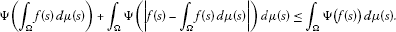(5.1)

It holds for all probability measure spaces $\left(\mathrm{\Omega },\mu \right)$ and all non-negative μ-integrable functions f on Ω if and only if $\mathrm{\Psi }:\left[0,\mathrm{\infty }\right)\to \mathbb{R}$ is a superquadratic function (see [, Theorem 2.3]).

The following theorem provides the main result of this section.

Theorem 5.1 Suppose that $\left({\mathrm{\Omega }}_{1},{\mathrm{\Sigma }}_{1},{\mu }_{1}\right)$, $\left({\mathrm{\Omega }}_{2},{\mathrm{\Sigma }}_{2},{\mu }_{2}\right)$, u, k, and K are as in Theorem  2.1, that the function $x↦u\left(x\right)\frac{k\left(x,y\right)}{K\left(x\right)}$ is integrable on ${\mathrm{\Omega }}_{1}$ for each fixed $y\in {\mathrm{\Omega }}_{2}$, and that the positive function v is defined by

$v\left(y\right)={\int }_{{\mathrm{\Omega }}_{1}}u\left(x\right)\frac{k\left(x,y\right)}{K\left(x\right)}\phantom{\rule{0.2em}{0ex}}d{\mu }_{1}\left(x\right)<\mathrm{\infty },\phantom{\rule{1em}{0ex}}y\in {\mathrm{\Omega }}_{2}.$

If Ψ is a superquadratic function on an interval $I\subseteq \left[0,\mathrm{\infty }\right)$ and $\mathrm{\Phi }:I\to \mathbb{R}$ is any function fulfilling (2.1) for some constants $0<{a}_{1}\le {a}_{2}<\mathrm{\infty }$, then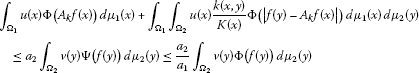(5.2)

holds for all measurable functions $f:{\mathrm{\Omega }}_{2}\to \mathbb{R}$ with values in I and for ${A}_{k}f$ defined on ${\mathrm{\Omega }}_{1}$ by (1.3).

Proof Applying inequality (5.1) to a superquadratic function Ψ, for each fixed $x\in {\mathrm{\Omega }}_{1}$, we get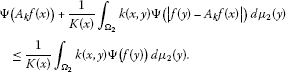Therefrom,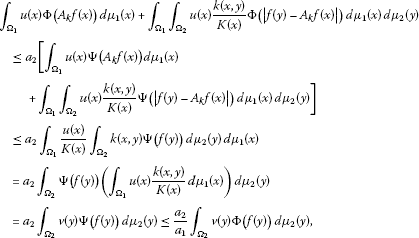so (5.2) is proved. □

As in previous sections, the above result can be specified for some usual measure spaces. Namely, suppose ${\mathrm{\Omega }}_{1}={\mathrm{\Omega }}_{2}={\mathbb{R}}_{+}^{n}$, $d{\mu }_{1}\left(\mathbf{x}\right)=d\mathbf{x}$, $d{\mu }_{2}\left(\mathbf{y}\right)=d\mathbf{y}$, and ${S}_{1}^{n}$ is defined by (4.2). Applying Theorem 5.1 to this setting and to $u\left(\mathbf{x}\right)$, $k\left(\mathbf{x},\mathbf{y}\right)$, and $v\left(\mathbf{y}\right)$ respectively replaced with $\frac{u\left(\mathbf{x}\right)}{{\mathbf{x}}^{\mathbf{1}}}{\chi }_{{S}_{1}^{n}}\left(\mathbf{x},\mathbf{x}\right)$, $k\left(\mathbf{x},\mathbf{y}\right){\chi }_{{S}_{1}^{n}}\left(\mathbf{x},\mathbf{y}\right)$, and $\frac{w\left(\mathbf{y}\right)}{{\mathbf{y}}^{\mathbf{1}}}$, we immediately obtain the following corollary.

Corollary 5.1 Let $\mathbf{0}<\mathbf{b}\le \mathrm{\infty }$, and let $k:\left(\mathbf{0},\mathbf{b}\right)×\left(\mathbf{0},\mathbf{b}\right)\to \mathbb{R}$ and $u:\left(\mathbf{0},\mathbf{b}\right)\to \mathbb{R}$ be non-negative measurable functions such that

$K\left(\mathbf{x}\right)={\int }_{\left(\mathbf{0},\mathbf{x}\right)}k\left(\mathbf{x},\mathbf{y}\right)\phantom{\rule{0.2em}{0ex}}d\mathbf{y}>0,\phantom{\rule{1em}{0ex}}\mathbf{x}\in \left(\mathbf{0},\mathbf{b}\right),$

and

$w\left(\mathbf{y}\right)={\mathbf{y}}^{\mathbf{1}}{\int }_{\left(\mathbf{y},\mathbf{b}\right)}u\left(\mathbf{x}\right)\frac{k\left(\mathbf{x},\mathbf{y}\right)}{K\left(\mathbf{x}\right)}\frac{d\mathbf{x}}{{\mathbf{x}}^{\mathbf{1}}}<\mathrm{\infty },\phantom{\rule{1em}{0ex}}\mathbf{y}\in \left(\mathbf{0},\mathbf{b}\right).$

If a real-valued function Ψ is superquadratic on an interval $I\subseteq \left[0,\mathrm{\infty }\right)$ and $\mathrm{\Phi }:I\to \mathbb{R}$ satisfies (2.1), then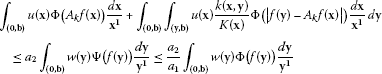(5.3)

holds for all measurable functions $f:\left(\mathbf{0},\mathbf{b}\right)\to \mathbb{R}$ with values in I and for ${A}_{k}f$ defined as

${A}_{k}f\left(\mathbf{x}\right)=\frac{1}{K\left(\mathbf{x}\right)}{\int }_{\left(\mathbf{0},\mathbf{x}\right)}k\left(\mathbf{x},\mathbf{y}\right)f\left(\mathbf{y}\right)\phantom{\rule{0.2em}{0ex}}d\mathbf{y},\phantom{\rule{1em}{0ex}}\mathbf{x}\in \left(\mathbf{0},\mathbf{b}\right).$

Remark 5.1 Observe that for $n=1$, $k\left(\mathbf{x},\mathbf{y}\right)\equiv 1$, and a superquadratic function Φ (that is, for ${a}_{1}={a}_{2}$), inequality (5.3) reduces to [, Proposition 2.1], so our result can be regarded as its generalization.

Analogously, applying Theorem 5.1 to $u\left(\mathbf{x}\right)$, $k\left(\mathbf{x},\mathbf{y}\right)$, and $v\left(\mathbf{y}\right)$ respectively replaced with $\frac{u\left(\mathbf{x}\right)}{{\mathbf{x}}^{\mathbf{1}}}{\chi }_{{S}_{2}^{n}}\left(\mathbf{x},\mathbf{x}\right)$, $k\left(\mathbf{x},\mathbf{y}\right){\chi }_{{S}_{2}^{n}}\left(\mathbf{x},\mathbf{y}\right)$, and $\frac{w\left(\mathbf{y}\right)}{{\mathbf{y}}^{\mathbf{1}}}$, where $\mathbf{0}\le \mathbf{b}<\mathrm{\infty }$ and ${S}_{2}^{n}=\left\{\left(\mathbf{x},\mathbf{y}\right)\in {\mathbb{R}}^{n}×{\mathbb{R}}^{n}:\mathbf{b}<\mathbf{x}\le \mathbf{y}<\mathrm{\infty }\right\}$, we get the following result dual to Corollary 5.1.

Corollary 5.2 Let $\mathbf{0}\le \mathbf{b}<\mathrm{\infty }$, and let $k:\left(\mathbf{b},\mathrm{\infty }\right)×\left(\mathbf{b},\mathrm{\infty }\right)\to \mathbb{R}$ and $u:\left(\mathbf{b},\mathrm{\infty }\right)\to \mathbb{R}$ be non-negative measurable functions such that

$\stackrel{˜}{K}\left(\mathbf{x}\right)={\int }_{\left(\mathbf{x},\mathrm{\infty }\right)}k\left(\mathbf{x},\mathbf{y}\right)\phantom{\rule{0.2em}{0ex}}d\mathbf{y}>0,\phantom{\rule{1em}{0ex}}\mathbf{x}\in \left(\mathbf{b},\mathrm{\infty }\right),$

and

$\stackrel{˜}{w}\left(\mathbf{y}\right)={\mathbf{y}}^{\mathbf{1}}{\int }_{\left(\mathbf{b},\mathbf{y}\right)}u\left(\mathbf{x}\right)\frac{k\left(\mathbf{x},\mathbf{y}\right)}{\stackrel{˜}{K}\left(\mathbf{x}\right)}\frac{d\mathbf{x}}{{\mathbf{x}}^{\mathbf{1}}}<\mathrm{\infty },\phantom{\rule{1em}{0ex}}\mathbf{y}\in \left(\mathbf{b},\mathrm{\infty }\right).$

If Ψ is a superquadratic function on an interval $I\subseteq \left[0,\mathrm{\infty }\right)$ and $\mathrm{\Phi }:I\to \mathbb{R}$ satisfies (2.1), then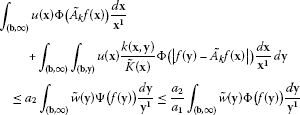(5.4)

holds for all measurable functions $f:\left(\mathbf{b},\mathrm{\infty }\right)\to \mathbb{R}$ with values in I and for $\stackrel{˜}{{A}_{k}}f$ defined by

$\stackrel{˜}{{A}_{k}}f\left(\mathbf{x}\right)=\frac{1}{\stackrel{˜}{K}\left(\mathbf{x}\right)}{\int }_{\left(\mathbf{x},\mathrm{\infty }\right)}k\left(\mathbf{x},\mathbf{y}\right)f\left(\mathbf{y}\right)\phantom{\rule{0.2em}{0ex}}d\mathbf{y},\phantom{\rule{1em}{0ex}}x\in \left(\mathbf{b},\mathrm{\infty }\right).$

Remark 5.2 For $n=1$, $k\left(\mathbf{x},\mathbf{y}\right)={\mathbf{y}}^{-2\mathbf{1}}$, and a superquadratic function Φ (that is, for ${a}_{1}={a}_{2}$), relation (5.4) reduces to [, Proposition 2.2]. Hence, our results can be seen as its generalization.

Finally, we apply Corollary 5.1 to the superquadratic function $\mathrm{\Psi }:\left[0,\mathrm{\infty }\right)\to \mathbb{R}$, $\mathrm{\Psi }\left(x\right)={x}^{p}$, where $p\ge 2$, and to some particular weights and kernels deducing a new class of multidimensional Hardy-type inequalities.

Example 5.1 Let $p,\gamma \in {\mathbb{R}}_{+}$, $p\ge 2$, and let $\mathbf{0}<\mathbf{b}\le \mathrm{\infty }$. Rewriting Corollary 5.1 with $I=\left[0,\mathrm{\infty }\right)$, $\mathrm{\Psi }\left(x\right)={x}^{p}$, $u\left(\mathbf{x}\right)\equiv 1$, and $k\left(\mathbf{x},\mathbf{y}\right)=\frac{{\gamma }^{n}}{{\mathbf{x}}^{\gamma \mathbf{1}}}{\left(\mathbf{x}-\mathbf{y}\right)}^{\left(\gamma -1\right)\mathbf{1}}{\chi }_{{S}_{1}^{n}}\left(\mathbf{x},\mathbf{y}\right)$, where ${S}_{1}^{n}$ is defined by (4.2), we get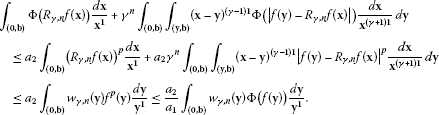(5.5)

As in Example 4.1, the operator ${R}_{\gamma ,n}$ is given by (4.4), while

${w}_{\gamma ,n}\left(\mathbf{y}\right)={\gamma }^{n}\prod _{i=1}^{n}B\left(1-\frac{{y}_{i}}{{b}_{i}};\gamma ,1\right)={\left(\mathbf{1}-\frac{\mathbf{y}}{\mathbf{b}}\right)}^{\gamma \mathbf{1}},\phantom{\rule{1em}{0ex}}\mathbf{y}\in \left(\mathbf{0},\mathbf{b}\right).$

Considering the second inequality in (5.5) with $\mathbf{k}\in {\mathbb{R}}^{n}$ such that $\frac{\mathbf{k}-\mathbf{1}}{p\mathbf{1}}>\mathbf{0}$ and with $\mathbf{c}=\left({b}_{1}^{\frac{{k}_{1}-1}{p}},\dots ,{b}_{n}^{\frac{{k}_{n}-1}{p}}\right)$ and $f\left({y}_{1}^{\frac{p}{{k}_{1}-1}},\dots ,{y}_{n}^{\frac{p}{{k}_{n}-1}}\right){\mathbf{y}}^{\frac{p\mathbf{1}}{\mathbf{k}-\mathbf{1}}-\mathbf{1}}$ instead of b and $f\left(\mathbf{y}\right)$ respectively, after a sequence of variable changes as in Example 4.1, we deduce the inequality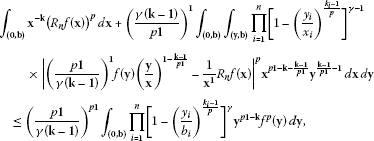(5.6)

where ${R}_{n}$ is defined by (4.6). Combining (5.5) and (5.6), we obtain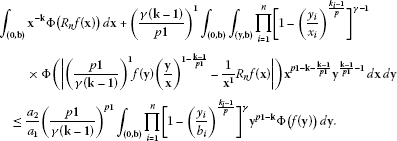(5.7)

Notice that our inequalities (5.6) and (5.7) generalize the results from  since inequality (5.6) reduces to [, Theorem 3.1] for $n=\gamma =1$.

A result dual to (5.7) generalizes the corresponding inequality from [, Theorem 3.2]. It is omitted since it can be deduced similarly, starting from Corollary 5.2.

## References

1. Krulić K, Pečarić J, Persson LE: Some new Hardy type inequalities with general kernels. Math. Inequal. Appl. 2009, 12: 473–485.

2. Lai Q: Weighted modular inequalities for Hardy type operators. Proc. Lond. Math. Soc. 1999, 79(3):649–672. 10.1112/S0024611599012010

3. Levinson N: Generalizations of an inequality of Hardy. Duke Math. J. 1964, 31: 389–394. 10.1215/S0012-7094-64-03137-0

4. Godunova EK: Generalization of a two-parameter Hilbert inequality. Izv. Vysš. Učebn. Zaved., Mat. 1967, 54(1):35–39. (Russian)

5. Oguntuase JA, Persson LE, Čižmešija A: Multidimensional Hardy-type inequalities via convexity. Bull. Aust. Math. Soc. 2008, 77: 245–260.

6. Oguntuase JA, Persson LE: Refinement of Hardy’s inequalities via superquadratic and subquadratic functions. J. Math. Anal. Appl. 2008, 339: 1305–1312. 10.1016/j.jmaa.2007.08.007

7. Čižmešija, A, Krulić, K, Pečarić, J: A new class of general refined Hardy-type inequalities with kernels. Rad Hrvat. Akad. Znan. Umjet. Mat. Znan. (2013, in press)

8. Čižmešija A, Pečarić J, Persson LE: On strengthened Hardy and Pólya-Knopp’s inequalities. J. Approx. Theory 2003, 125: 74–84. 10.1016/j.jat.2003.09.007

9. Kaijser S, Nikolova L, Persson LE, Wedestig A: Hardy type inequalities via convexity. Math. Inequal. Appl. 2005, 8(3):403–417.

10. Andersen KF: Weighted inequalities for the Stieltjes transformation and Hilbert’s double series. Proc. R. Soc. Edinb. A 1980, 86(1–2):75–84. 10.1017/S0308210500012014

11. Shum DT: On integral inequalities related to Hardy’s. Can. Math. Bull. 1971, 14(2):225–230.

12. Goyal SP, Parashar A: Some results for unified Riemann-zeta function. Kyungpook Math. J. 2002, 42(1):87–94.

13. Abramovich S, Banić S, Matić M: Superquadratic functions in several variables. J. Math. Anal. Appl. 2007, 137(2):1444–1460.

14. Abramovich S, Jameson G, Sinnamon G: Refining of Jensen’s inequality. Bull. Math. Soc. Sci. Math. Roum. 2004, 47(95):3–14.

15. Abramovich S, Jameson G, Sinnamon G: Inequalities for averages of convex and superquadratic functions. J. Inequal. Pure Appl. Math. 2004., 7(2): Article ID 70

## Acknowledgements

The third author expresses his gratitude to the Abdus Salam International Centre for Theoretical physics, Trieste, Italy, for financial support to carry out this work within the framework of the associate scheme of the Centre. The research of the second and fifth authors was supported by the Croatian Ministry of Science, Education and Sports, under the Research Grants 058-1170889-1050 (second author) and 082-0000000-0893 (fifth author). All authors express their gratitude to the careful referee whose advices improved the final version of this paper.

## Author information

Authors

### Competing interests

The authors declare that they have no competing interests.

### Authors’ contributions

All authors contributed equally to the research and the way its results are presented in this paper. All authors read and approved the final manuscript.

## Rights and permissions

Reprints and Permissions

Adeleke, E., Čižmešija, A., Oguntuase, J. et al. On a new class of Hardy-type inequalities. J Inequal Appl 2012, 259 (2012). https://doi.org/10.1186/1029-242X-2012-259

• Accepted:

• Published:

• DOI: https://doi.org/10.1186/1029-242X-2012-259

### Keywords

• Hardy inequality
• power weights
• convex functions